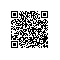# 算法步骤

#include<iostream>

using namespace std;

//选择排序，参数为数组和数组长度

void selectionSort(int arr[],int n){

for(int i=0;i<n;i++){

//找到[i,n)范围内的最小值坐标，初始最小值坐标为i

int minIndex=i;

for(int j=i+1;j<n;j++){

if(arr[j]<arr[minIndex])

minIndex=j;

}

//交换坐标值

swap(arr[i],arr[minIndex]);

}

}

int main(){

int a={10,9,8,7,6,5,4,3,2,1};

selectionSort(a,10);

for(int i=0;i<10;i++)

cout << a[i] << " ";

cout << endl;

return 0;

}

//输出

//1 2 3 4 5 6 7 8 9 10

//选择排序

template<typename T>

void selectionSort(T arr[],int n){

for(int i=0;i<n;i++){

//找到[i,n)范围内的最小值坐标，初始最小值坐标为i

int minIndex=i;

for(int j=i+1;j<n;j++){

if(arr[j]<arr[minIndex])

minIndex=j;

}

//交换坐标值

swap(arr[i],arr[minIndex]);

}

}

## 操作符重载

//Student.h

#ifndef STUDENT_H

#define STUDENT_H

#include<iostream>

#include<string>

using namespace std;

struct Student{

string name;

int score;

bool operator < (const Student& otherStudent){

return this->score<otherStudent.score

}

//重构operator<<

friend ostream& operator << (ostream& os,const Student& student){

os<<"Student:"<<student.name<<" "<<student.score<<endl;

return os;

}

};

#endif

//selectionSort.cpp

#include<iostream>

#include "Student.h"//引入Student.h

using namespace std;

//选择排序

template<typename T>

void selectionSort(T arr[],int n){

for(int i=0;i<n;i++){

//找到[i,n)范围内的最小值坐标，初始最小值坐标为i

int minIndex=i;

for(int j=i+1;j<n;j++){

if(arr[j]<arr[minIndex])

minIndex=j;

}

//交换坐标值

swap(arr[i],arr[minIndex]);

}

}

int main(){

Student d={ {"D",90},{"C",100},{"B",95},{"A",95} };

selectionSort(d,4);

for(int i=0;i<4;i++)

cout << d[i];

cout<<endl;

return 0;

}

/*从小到大排序

Student:D 90

Student:B 95

Student:A 95

Student:C 100

*/

### 成员函数

this：是一个pointer（指针），指向函数调用者，即对象。（谁调用我，我就指向谁）

//  ostream& operator << (this,ostream& os)

//                  ||

ostream& operator << (ostream& os){

os<<"Student:"<<this->name<<" "<<(*this).score<<endl;

return os;

}

//d[i]为一个对象，对象调用operator<<函数，参数为cout

d[i].operator <<(cout);

### 全局函数

friend ostream& operator << (ostream& os,const Student& stu){

os<<"Student:"<<stu.name<<" "<<stu.score<<endl;

return os;

}

//全局函数直接调用，参数为cout和Student的对象d[i]

operator<<(cout,d[i]);

//与下行代码效果相同

cout << d[i];使用钉钉扫一扫加入圈子
+ 订阅